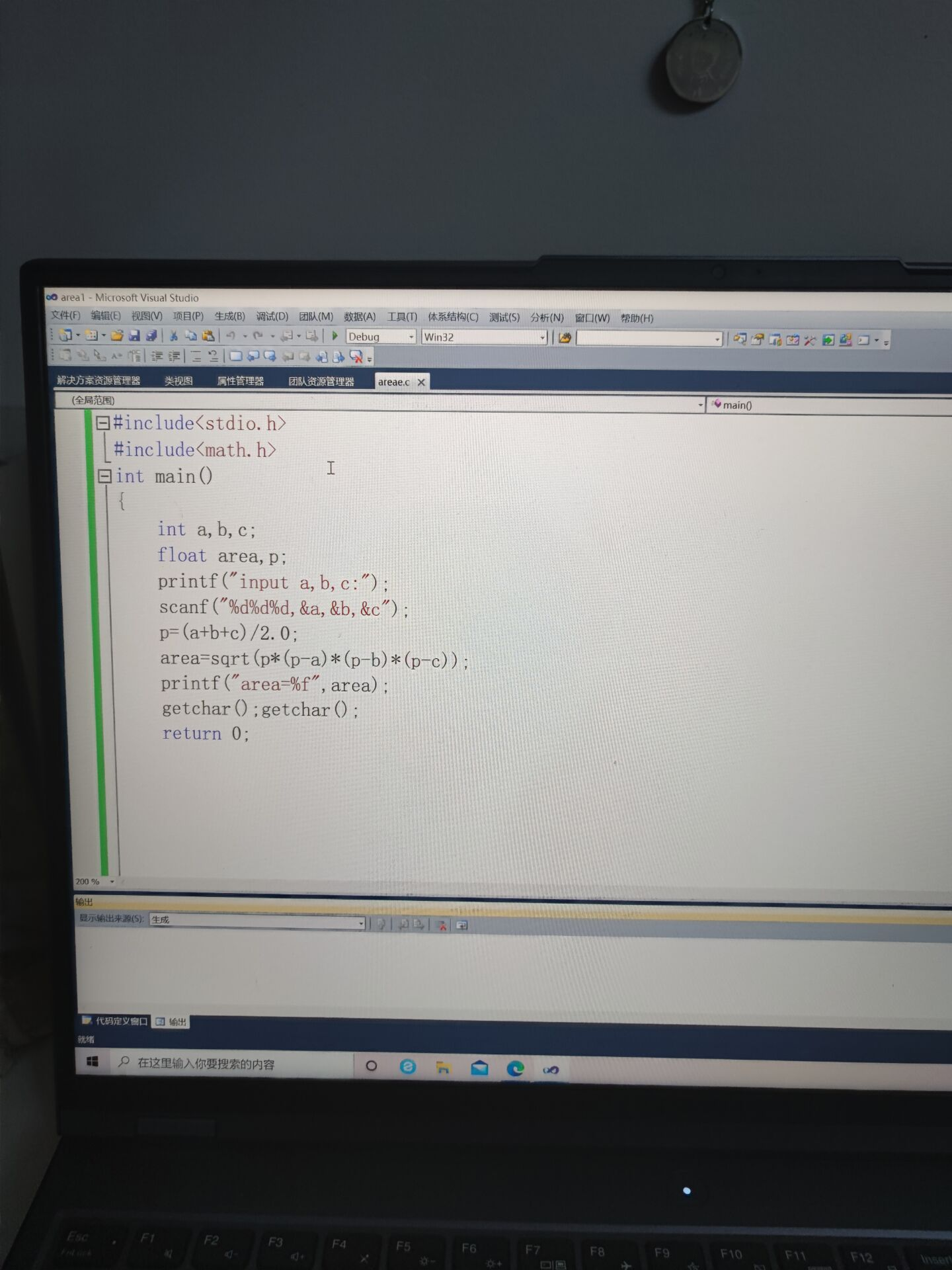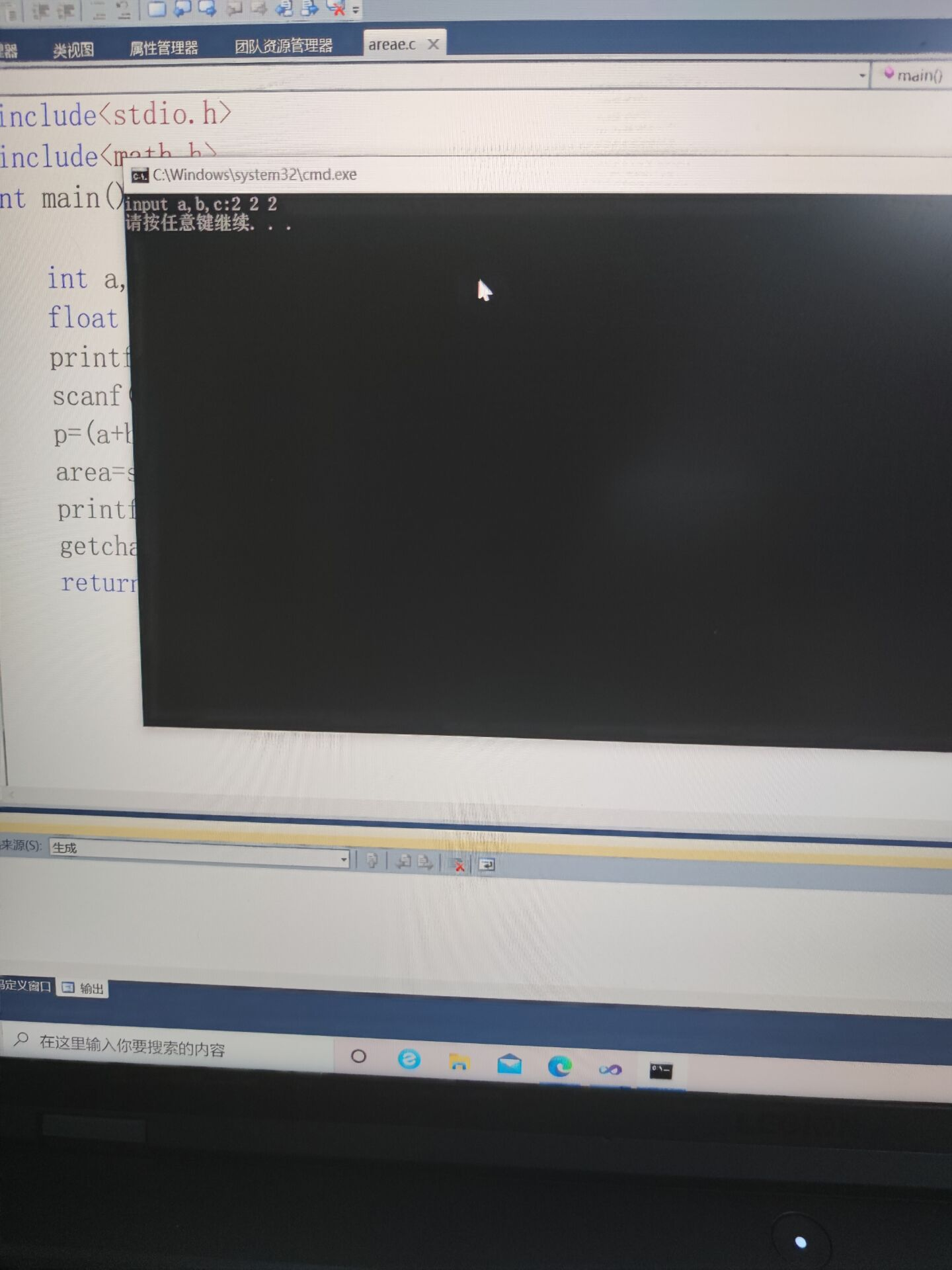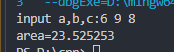2021-09-21 20:57

# 只能输入数据，没有计算结果• 收藏

#### 1条回答默认 最新

•已采纳

你&a,&b,&c不要写在引号里面
正确的是

``````    scanf("%d%d%d",&a,&b,&c);
``````

你题目的解答代码如下：（如有帮助，望采纳！谢谢! 点击我这个回答右上方的【采纳】按钮）

``````#include<stdio.h>
#include<math.h>
int main(){
int a,b,c;
float area,p;
printf("input a,b,c:");
scanf("%d%d%d",&a,&b,&c);
p=(a+b+c)/2.0;
area=sqrt(p*(p-a)*(p-b)*(p-c));
printf("area=%f",area);
getchar();getchar();
return 0;
}
``````已采纳该答案
打赏 评论